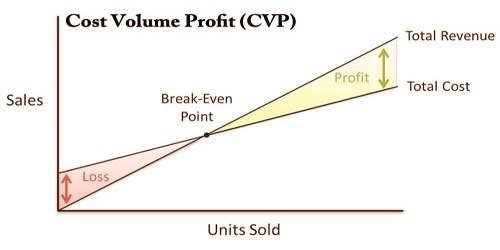Accounting

# Cost Volume Profit (CVP)Cost-volume-profit (CVP) is a cost accounting approach that looks at the effect on operating profit that different rates of cost and volume have. A cost volume profit (CVP) chart could be a graphical representation of the cost-volume-profit analysis. In other words, it is a graph showing the relation between the unit prices produced and also the number of units produced using fixed costs, operating costs, and net sales. CVP could be a simplified model, useful for elementary instruction, and for short-run decisions.

The cost-volume-profit (CVP) analysis, also widely known as a break-even analysis, tries to calculate the break-even point for various sales volumes and cost structures and can be useful for managers making short-term economic decisions. An organization will experience no profit or loss at this break-even stage. This break-even point could be an initial exam that precedes a more thorough review of the CVP.

The cost-volume-profit (CVP) model includes many assumptions, including a constant sales price, fixed costs, and variable cost per product. Running this study involves using several prices, cost, and other variables equations, and then drawing them out on an economic graph.

The components of CVP analysis are:

• Level or volume of activity.
• Unit selling prices
• Variable cost per unit
• Total fixed costs
• Manpower Cost Direct and indirect

The CVP formula can be used to measure the sales volume needed to cover costs and even split the sales volume formula in the CVP breakeven, as follows:

Breakeven Sales Volume = FC/CM

Where:

FC = Fixed costs

CM = Contribution margin = Sales − Variable Costs

The assumptions of the CVP model yield the following linear equations for total costs and total revenue (sales):

Total costs = fixed costs + (unit variable cost × number of units)

Total revenue = sales price × number of unit

These are linear thanks to the assumptions of constant costs and costs, and there’s no distinction between units produced and units sold, as these are assumed to be equal. CVP analysis is just reliable if costs are fixed within a specified production level. All units produced are assumed to be sold, and everyone fixed costs must be stable in CVP analysis.

​Analyzing the CVP also controls the margin for drug participation. The margin of profit is the difference between net revenue and the total expense of the product. CVP simplifies breakeven calculation in break-even analysis, and more generally make easy target revenue sales calculation. It simplifies the analysis of operational decisions on short-run trade-offs.

Information Sources: